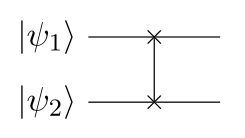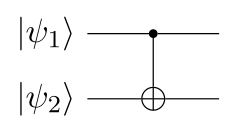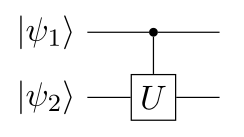Categories: Quantum information.

# Quantum gate

In quantum computing, quantum gates are the equivalent of classical binary logic gates such as $$\mathrm{NOT}$$, $$\mathrm{AND}$$, etc. Because of the continuous nature of qubits, the number of possible quantum gates is uncountably infinite, so we only consider the most important examples here.

## One-qubit gates

As an example, consider the following must general single-qubit state $$\ket{\psi}$$:

\begin{aligned} \ket{\psi} = \alpha \ket{0} + \beta \ket{1} = \begin{bmatrix} \alpha \\ \beta \end{bmatrix} \end{aligned}

Arguably the most famous and/or most fundamental quantum gates are the Pauli matrices:

\begin{aligned} \boxed{ X = \begin{bmatrix} 0 & 1 \\ 1 & 0 \end{bmatrix} } \qquad \boxed{ Y = \begin{bmatrix} 0 & -i \\ i & 0 \end{bmatrix} } \qquad \boxed{ Z = \begin{bmatrix} 1 & 0 \\ 0 & -1 \end{bmatrix} } \end{aligned}

They have the following effect on $$\ket{\psi}$$. Note that $$X$$ is equivalent to the classical $$\mathrm{NOT}$$ gate (and is often given that name), and $$Z$$ is sometimes called the phase-flip gate:

\begin{aligned} X \ket{\psi} = \begin{bmatrix} \beta \\ \alpha \end{bmatrix} \qquad Y \ket{\psi} = \begin{bmatrix} -i \beta \\ i \alpha \end{bmatrix} \qquad Z \ket{\psi} = \begin{bmatrix} \alpha \\ -\beta \end{bmatrix} \end{aligned}

In fact, $$Z$$ is a specific case of the phase shift gate $$R_\phi$$, which modifies the qubit’s phase without changing its amplitudes. For an angle $$\phi$$, it is given by:

\begin{aligned} \boxed{ R_\phi = \begin{bmatrix} 1 & 0 \\ 0 & e^{i \phi} \end{bmatrix} } \end{aligned}

For $$\phi = \pi$$, we recover the Pauli-$$Z$$ gate. In general, the action of $$R_\phi$$ is as follows:

\begin{aligned} R_\phi \ket{\psi} = \begin{bmatrix} \alpha \\ e^{i \phi} \beta \end{bmatrix} \end{aligned}

Two common special cases of $$R_\phi$$ are $$\phi = \pi/2$$ and $$\phi = \pi/4$$, respectively called $$S$$ and $$T$$:

\begin{aligned} \boxed{ S = R_{\pi/2} = \begin{bmatrix} 1 & 0 \\ 0 & i \end{bmatrix} } \qquad \quad \boxed{ T = R_{\pi/4} = \frac{1}{\sqrt{2}} \begin{bmatrix} \sqrt{2} & 0 \\ 0 & 1 + i \end{bmatrix} } \end{aligned}

Finally, we have the Hadamard gate $$H$$, which is defined as follows:

\begin{aligned} \boxed{ H = \frac{1}{\sqrt{2}} \begin{bmatrix} 1 & 1 \\ 1 & -1 \end{bmatrix} } \end{aligned}

Its action consists of rotating the qubit by $$\pi$$ around the axis $$(X + Z) / \sqrt{2}$$ of the Bloch sphere:

\begin{aligned} H \ket{\psi} = \frac{1}{\sqrt{2}} \begin{bmatrix} \alpha + \beta \\ \alpha - \beta \end{bmatrix} \end{aligned}

Notably, it maps the eigenstates of $$X$$ and $$Z$$ to each other, and is its own inverse (i.e. unitary):

\begin{aligned} H \ket{0} = \ket{+} \qquad H \ket{1} = \ket{-} \qquad H \ket{+} = \ket{0} \qquad H \ket{-} = \ket{1} \end{aligned}

The Clifford gates are a set including $$X$$, $$Y$$, $$Z$$, $$H$$ and $$S$$, or more generally any gates that rotate by multiples of $$\pi/2$$ around the Bloch sphere. This set is not universal, meaning that if we start from $$\ket{0}$$, we can only reach $$\ket{0}$$, $$\ket{1}$$, $$\ket{+}$$, $$\ket{-}$$, $$\ket{+i}$$ $$\ket{-i}$$ using these gates.

If we add any non-Clifford gate, for example $$T$$, then we can reach any point on the Bloch sphere, which means that the set is universal.

However, there is a problem: a qubit has an uncountable infinity of states, but a quantum circuit consists of a countably infinite sequence of gates, at most. Therefore, technically, we can never reach the whole Bloch sphere, but we can come up with circuits that approximate a target state to some degree $$\varepsilon$$. This is the definition of universality: any state can be approximated.

## Two-qubit gates

As an example, let us consider the following two pure one-qubit states $$\ket{\psi_1}$$ and $$\ket{\psi_2}$$:

\begin{aligned} \ket{\psi_1} = \alpha_1 \ket{0} + \beta_1 \ket{1} = \begin{bmatrix} \alpha_1 \\ \beta_1 \end{bmatrix} \qquad \quad \ket{\psi_2} = \alpha_2 \ket{0} + \beta_2 \ket{1} = \begin{bmatrix} \alpha_2 \\ \beta_2 \end{bmatrix} \end{aligned}

The composite state of both qubits, assuming they are pure, is then their tensor product $$\otimes$$:

\begin{aligned} \ket{\psi_1 \psi_2} = \ket{\psi_1} \otimes \ket{\psi_2} &= \alpha_1 \alpha_2 \ket{00} + \alpha_1 \beta_2 \ket{01} + \beta_1 \alpha_2 \ket{10} + \beta_1 \beta_2 \ket{11} \\ &= c_{00} \ket{00} + c_{01} \ket{01} + c_{10} \ket{10} + c_{11} \ket{11} \end{aligned}

Note that a two-qubit system may be entangled, in which case the coefficients $$c_{00}$$ etc. cannot be written as products, i.e. $$\ket{\psi_2}$$ cannot be expressed separately from $$\ket{\psi_1}$$, and vice versa.

In other words, the general action of a two-qubit quantum gate can be expressed in the basis of $$\ket{00}$$, $$\ket{01}$$, $$\ket{10}$$ and $$\ket{11}$$, but not always in the basis of $$\ket{0}_1$$, $$\ket{1}_1$$, $$\ket{0}_2$$ and $$\ket{1}_2$$.

With that said, the first two-qubit gate is $$\mathrm{SWAP}$$, which simply swaps $$\ket{\psi_1}$$ and $$\ket{\psi_2}$$:\begin{aligned} \boxed{ \mathrm{SWAP} = \begin{bmatrix} 1 & 0 & 0 & 0 \\ 0 & 0 & 1 & 0 \\ 0 & 1 & 0 & 0 \\ 0 & 0 & 0 & 1 \end{bmatrix} } \end{aligned}

This matrix is given in the basis of $$\ket{00}$$, $$\ket{01}$$, $$\ket{10}$$ and $$\ket{11}$$. Note that $$\mathrm{SWAP}$$ cannot generate entanglement, so if its input is separable, its output is too. In any case, its effect is clear:

\begin{aligned} \mathrm{SWAP} \ket{\psi_1 \psi_2} &= c_{00} \ket{00} + c_{10} \ket{01} + c_{01} \ket{10} + c_{11} \ket{11} \end{aligned}

Next, there is the controlled NOT gate $$\mathrm{CNOT}$$, which “flips” (applies $$X$$ to) $$\ket{\psi_2}$$ if $$\ket{\psi_1}$$ is true:\begin{aligned} \boxed{ \mathrm{CNOT} = \begin{bmatrix} 1 & 0 & 0 & 0 \\ 0 & 1 & 0 & 0 \\ 0 & 0 & 0 & 1 \\ 0 & 0 & 1 & 0 \end{bmatrix} } \end{aligned}

That is, it swaps the last two coefficients $$c_{10}$$ and $$c_{11}$$ in the composite state vector:

\begin{aligned} \mathrm{CNOT} \ket{\psi_1 \psi_2} &= c_{00} \ket{00} + c_{01} \ket{01} + c_{11} \ket{10} + c_{10} \ket{11} \end{aligned}

More generally, from every one-qubit gate $$U$$, we can define a two-qubit controlled U gate $$\mathrm{CU}$$, which applies $$U$$ to $$\ket{\psi_2}$$ if $$\ket{\psi_1}$$ is true:\begin{aligned} \boxed{ \mathrm{CU} = \begin{bmatrix} 1 & 0 & 0 & 0 \\ 0 & 1 & 0 & 0 \\ 0 & 0 & u_{00} & u_{01} \\ 0 & 0 & u_{10} & u_{11} \end{bmatrix} } \end{aligned}

Where the lower-right 2x2 block is simply $$U$$. The general action of this gate is given by:

\begin{aligned} \mathrm{CU} \ket{\psi_1 \psi_2} &= c_{00} \ket{00} + c_{01} \ket{01} + (c_{10} u_{00} + c_{11} u_{01}) \ket{10} + (c_{10} u_{10} + c_{11} u_{11}) \ket{11} \end{aligned}

A set of gates is universal if all possible mappings from $$n$$ to $$n$$ qubits can be approximated using only these gates. A minimal universal set is $$\{\mathrm{CNOT}, T, S\}$$, and there exist many others.

1. J.S. Neergaard-Nielsen, Quantum information: lectures notes, 2021, unpublished.
2. S. Aaronson, Introduction to quantum information science: lecture notes, 2018, unpublished.